Anzeige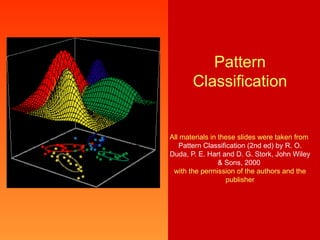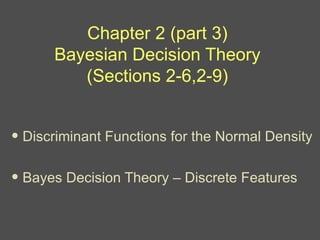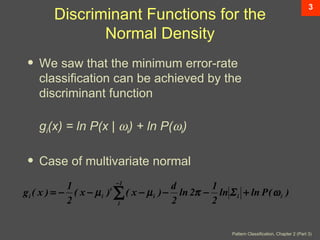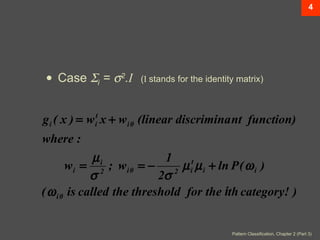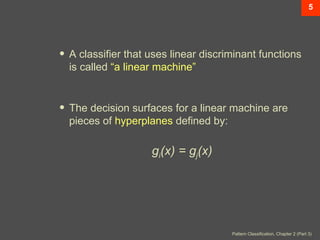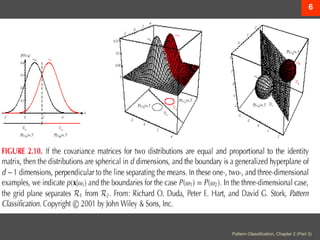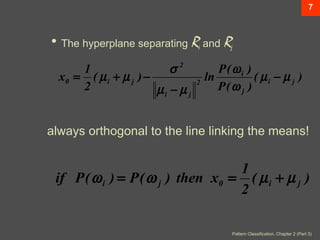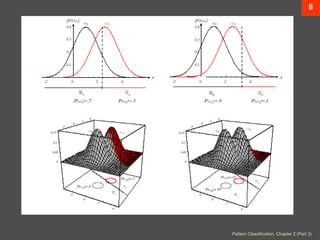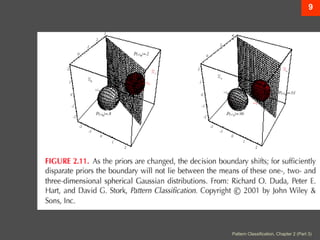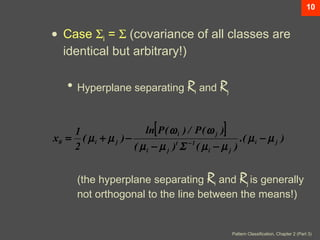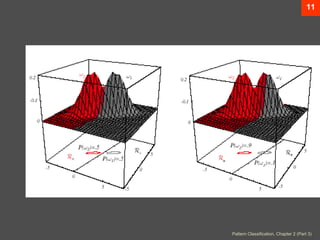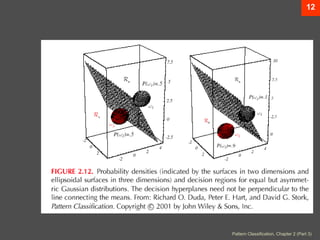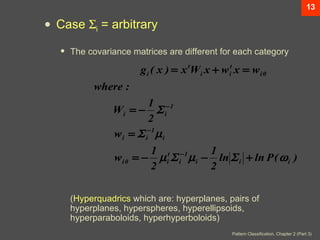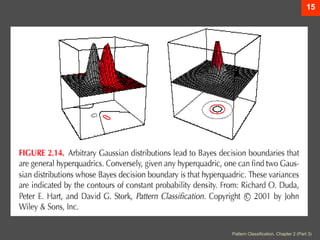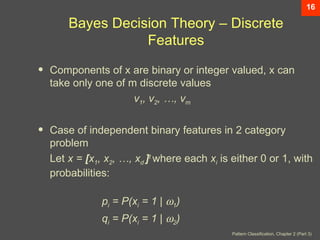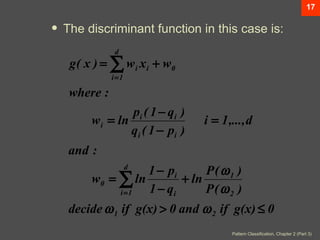1 von 17
Anzeige

### Pr1

1. Pattern Classification All materials in these slides were taken from Pattern Classification (2nd ed) by R. O. Duda, P. E. Hart and D. G. Stork, John Wiley & Sons, 2000 with the permission of the authors and the publisher
2. Chapter 2 (part 3) Bayesian Decision Theory (Sections 2-6,2-9) • Discriminant Functions for the Normal Density • Bayes Decision Theory – Discrete Features
3. 3 Discriminant Functions for the Normal Density • We saw that the minimum error-rate classification can be achieved by the discriminant function gi(x) = ln P(x | ωi) + ln P(ωi) • Case of multivariate normal −1 1 d 1 g i ( x ) = − ( x − µ i ) ∑ ( x − µ i ) − ln 2π − ln Σ i + ln P ( ω i ) t 2 i 2 2 Pattern Classification, Chapter 2 (Part 3)
4. 4 • Case Σi = σ2.I (I stands for the identity matrix) g i ( x ) = w it x + w i 0 (linear discrimina nt function) where : µi 1 wi = 2 ; wi 0 = − µ it µ i + ln P ( ω i ) σ 2σ 2 ( ω i 0 is called the threshold for the ith category! ) Pattern Classification, Chapter 2 (Part 3)
5. 5 • A classifier that uses linear discriminant functions is called “a linear machine” • The decision surfaces for a linear machine are pieces of hyperplanes defined by: gi(x) = gj(x) Pattern Classification, Chapter 2 (Part 3)
6. 6 Pattern Classification, Chapter 2 (Part 3)
7. 7 • The hyperplane separating R and R i j 1 σ2 P( ωi ) x0 = ( µ i + µ j ) − ln ( µi − µ j ) 2 µi − µ j 2 P( ω j ) always orthogonal to the line linking the means! 1 if P ( ω i ) = P ( ω j ) then x0 = ( µ i + µ j ) 2 Pattern Classification, Chapter 2 (Part 3)
8. 8 Pattern Classification, Chapter 2 (Part 3)
9. 9 Pattern Classification, Chapter 2 (Part 3)
10. 10 • Case Σ = Σ (covariance of all classes are i identical but arbitrary!) • Hyperplane separating R and R i j 1 x0 = ( µ i + µ j ) − [ ] ln P ( ω i ) / P ( ω j ) .( µ i − µ j ) 2 ( µi − µ j ) Σ ( µi − µ j ) t −1 (the hyperplane separating Ri and Rj is generally not orthogonal to the line between the means!) Pattern Classification, Chapter 2 (Part 3)
11. 11 Pattern Classification, Chapter 2 (Part 3)
12. 12 Pattern Classification, Chapter 2 (Part 3)
13. 13 • Case Σ = arbitrary i • The covariance matrices are different for each category g i ( x ) = x tW i x + w it x = w i 0 where : 1 −1 Wi = − Σ i 2 w i = Σ i−1 µ i 1 t −1 1 w i 0 = − µ i Σ i µ i − ln Σ i + ln P ( ω i ) 2 2 (Hyperquadrics which are: hyperplanes, pairs of hyperplanes, hyperspheres, hyperellipsoids, hyperparaboloids, hyperhyperboloids) Pattern Classification, Chapter 2 (Part 3)
14. 14 Pattern Classification, Chapter 2 (Part 3)
15. 15 Pattern Classification, Chapter 2 (Part 3)
16. 16 Bayes Decision Theory – Discrete Features • Components of x are binary or integer valued, x can take only one of m discrete values v1, v2, …, vm • Case of independent binary features in 2 category problem Let x = [x1, x2, …, xd ]t where each xi is either 0 or 1, with probabilities: pi = P(xi = 1 | ω1) qi = P(xi = 1 | ω2) Pattern Classification, Chapter 2 (Part 3)
17. 17 • The discriminant function in this case is: d g ( x ) = ∑ w i x i + w0 i =1 where : pi ( 1 − q i ) w i = ln i = 1 ,..., d q i ( 1 − pi ) and : 1 − pi d P( ω1 ) w0 = ∑ ln + ln i =1 1 − qi P( ω 2 ) decide ω 1 if g(x) > 0 and ω 2 if g(x) ≤ 0 Pattern Classification, Chapter 2 (Part 3)
Anzeige